# AP Computer Science A : Int

## Example Questions

### Example Question #1 : Int

Consider the following code:

int i = 55, j = 3;

System.out.println((i / j + 3) / 5);

What is the output for the code above?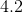There is an error in the code.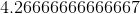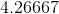Explanation:

Be careful! This is integer division.  Therefore, for every division, you will lose your decimal place.  Thus:

i / j is the same as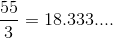, but it becomes.

Then, you will have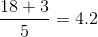.  Once more, you lose the decimal and getas your result.

### Example Question #1 : Primitive Data Types

Which of the following gurantees that your division does not lose its decimal portion?

double d = 55 / 3;

None of the answers are correct.

double d = 1.0 * 55 / 3;

double d = (double)((int)55 / (int) 3);

double d = (double)(55/3);

double d = 1.0 * 55 / 3;

Explanation:

Remember that so long as one element of a set of multiplications / divisions is a floating point value, the whole thing will be a floating point value.  Thus, the correct answer is:

double d = 1.0 * 55 / 3;

You have to evaluate the right side:

1.0 * 55 will become 55.0.  This will then finish out as a double in the division and store that value in d.

Note that the following does not work:

double d = (double)(55 / 3);

This code will first do the integer division 55 / 3.  This will resolve to 18.  Only then will the double cast occur.  This will give you 18.0 but not 18.33333...

### Example Question #4 : Standard Data Structures

Consider the following code:

int i = 100;

double d = 4.55, d2 = 3.75;

int j = (int)(d * 100 + d2);

What is the value of j at the end of the code's execution?

399

458

459

403

411

458

Explanation:

Do not over think this.  Begin by evaluating the expression:

d * 100 + d2

This is the same thing as: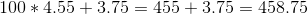Now, this value is then cast to an integer:

(int)(458.75)

Remember that when you type cast an integer from a double value, you drop the decimal place completely.  You do not round up or down.  You just truncate it off.  Thus, the answer is 458.

### Example Question #5 : Standard Data Structures

Consider the following code:

int i = 3;

for(int j = 5; j > 0; j--) {

i += i;

}

What will be the value of i at the end of this loop's iteration?

96

243

729

24

48

96

Explanation:

The loop in question executes for j values of 5 through 1.  Thus, you will execute 5 times.  For each looping, then you will have:

i = 3 + 3 = 6

i = 6 + 6 = 12

i = 12 + 12 = 24

i = 24 + 24 = 48

i = 48 + 48 = 96

### Example Question #6 : Standard Data Structures

Consider the code below:

int i = 5, p = 27;

for(int l = 23; l < p; l++) {

     i *= (l - 22);

}

What is the value for i at the end of the code above?

75

120

5

0

150

120

Explanation:

You could always trace the loop in the code manually. You know that it is going to run from l = 23 to l = 26.  Recall that *= could be rewritten:

i = i * (l - 22)

Now, let's consider our first looping. For this, we would have:

i = 5 * (23 - 22) = 5 * 1

Now, let's calculate i for each looping from 23 to 26:

23: 5

24: 5 * (24 - 22) = 5 * 2 = 10

25: 10 * (25 - 22) = 10 * 3 = 30

26: 30 * (26 - 22) = 30 * 4 = 120

### Example Question #7 : Standard Data Structures

Consider the code below:

int val = 205;

for(int i = 0; i < 5; i++) {

val /= 2;

}

At the end of its execution, what is the value of the variable val in the code above?

25

6

6.40625

5.90225

12

6

Explanation:

Recall that the operator /= could be rewritten:

val = val / 2;

Now, recall also that integer division drops the decimal portion always. Therefore, this program will loop 5 times, doing the division of val by 2 each time. This gives you: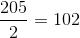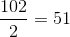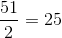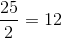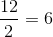### Example Question #8 : Standard Data Structures

Which of the following variable assignments is NOT a valid assignment statement?

a. 15 = x - 15;

b. num = x * y;

c. x = y + 15

d. x + y = 15

d, c

b, c

a, d

d

a, d

Explanation:

a. 15 = x - 15;

You can not assign a value to an integer because they are not variables and do not store information.

d. x + y = 15

This statement does not assign a value to a single integer, therefore, it is not a valid variable assignment.

### Example Question #1 : Int

Refer to the following line of code:

double d = -4.73;

Which of the following correctly rounds d to the nearest integer?

int roundedNumber = (int) Math.abs(d);

int roundedNumber = (int) (d+.5)

int roundedNumber = Math.abs(d);

int roundedNumber = Math.abs((int) (d))

int roundedNumber = (int) (d - 0.5)

int roundedNumber = (int) (d - 0.5)

Explanation:

The answer is (int) (d - 0.5) because you want roundNumber to be set to -5, and casting a negative number of type double to int will round the number up to the greater integer.

Math.abs(d) returns the absolute value of d, and does not typecast to int. This option will throw an incompatible types compiler error.

If you add the (int) cast to Math.abs(d), you will floor the number, or round down d to 4.

The statement int roundedNumber = (int) (d+.5) will add .5 to -4.67 resulting in (int) -4.17. This will result in roundedNumber being set to -4, since casting a negative double to an int will actually do a cieling operation ( for example (int) -4.99 is still -4).

Finally, int roundedNumber = Math.abs((int) (d)) casts d to -4 and the takes the absolute value of -4, resulting in 4.

### Example Question #2 : Int

Suppose you are given the following lines of code:

Integer a = new Integer(10);

Integer b = new Integer(4);

Which of the following lines will not generate an error?

I. if (a.intValue() == b.intValue())

II if ((a.toString()).equals(b.toString()))

III if ((a.intValue()).equals(b.intValue()))

I

I and II

II

III

I, II, III